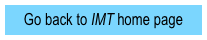Tutorials on vector force fields

Conservative forces and equipotential diagramsPretest (.pdf)

Tutorial [topo] (.pdf)

Tutorial [elec] (.pdf)

Homework (.pdf)

Exam questions (.docx)

Instr. notes [topo] (.pdf)

Instr. notes [elec] (.pdf)

All materials (~3.1MB .zip,

.docx and .pdf)

Tutorial title

Emphasis (what do students do?)

Relate magnitude of force to spatial rate-of-change of potential energy (separate versions of tutorial focus on topographic maps and electric potential diagrams)

Operationally define gradient of a scalar field; apply definition to relationship between force and potential energy

Conservative
force fieldsContact information for co-PIs

Department of Physics

Grand Valley State University

Allendale, MI 49401

Tel.:  616-331-2524

FAX:  616-331-3740

Email:  ambroseb@gvsu.edu

Michael C. Wittmann

Department of Physics and Astronomy

5709 Bennett Hall

University of Maine

Orono, ME  04401-5709

Tel.:  207-581-1237

FAX:  207-581-3410

Email:  wittmann@umit.maine.edu

Connect path integral of forces to work done

by those forces; articulate properties of conservative forces in terms of path integrals

Operationally define vector curl; apply definition to conservative force fieldsSeparating forces

Pretest (.pdf)

Tutorial (.pdf)

Homework (.pdf)

All materials (~650KB .zip,

.docx and .pdf)

Pretest (.pdf)

Tutorial (.pdf)

Homework (.pdf)

Exam questions (.docx)

Instructor notes (.pdf)

All materials (~2.6MB .zip,

.docx and .pdf)

Operationally define separability of vector forces, using both force field diagrams and vector calculus

Distinguish between separability and conservation

Sponsored in part by NSF grants DUE-0441426 and DUE-0442388Intermediate Mechanics Tutorials are modeled after:

1. Tutorials in Introductory Physics, L.C. McDermott P.S. Shaffer, and the Physics Education Group at the University of Washington (Prentice Hall, 2002)

1. Activity-Based Tutorials, M.C. Wittmann, R.N. Steinberg, E.F. Redish, and the University of Maryland Physics Education Research Group (Wiley, 2004 and 2005)